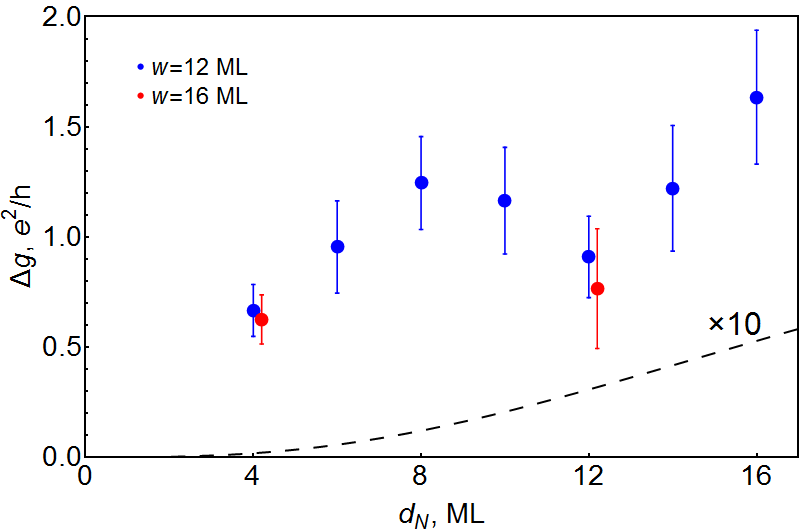Examples

# Density-functional Description of Spin Orbit Torque

Interfacial contribution to spin-orbit torque and magnetoresistance in ferromagnet/heavy-metal bilayers

Magnetization in nanoelectronic devices can be manipulated by angular momentum transfer. A spin-orbit torque (SOT) is a manifestation of such transfer driven by spin-orbit coupling in ferromagnet/heavy-metal (FM/HM) bilayers, which is also responsible for anisotropic transport properties such as spin-Hall magnetoresistance (SMR). SOT and SMR involve complex processes both in the bulk and at the interface. The role played by spin currents in angular momentum transfer is still being actively investigated both experimentally and theoretically. However, it is also clear that SOT is not fully determined by spin currents because magnetization can exchange angular momentum with the lattice.

In a recent paper, the thickness dependence of spin-orbit torque and magnetoresistance in ferromagnet/heavy-metal bilayers is studied using the first-principles non-equilibrium Green’s function formalism combined with the Anderson disorder model. A systematic expansion in orthogonal vector spherical harmonics is used for the angular dependence of the torque. The damping-like torque in Co/Pt and Co/Au bilayers can be described as a sum of the spin-Hall contribution, which increases with thickness in agreement with the spin-diffusion model, and a comparable interfacial contribution. The magnetoconductance in the plane perpendicular to the current in Co/Pt bilayers is of the order of a conductance quantum per interfacial atom, exceeding the prediction of the spin-Hall model by more than an order of magnitude. This suggests that the spin-Hall magnetoresistance,’’ similarly to the damping-like torque, has a large interfacial contribution unrelated to the spin-Hall effect.Figure: Dependence of the magnetoconductances $\Delta_{yx}g$ (red symbols) and $\Delta_{yz}g$ (blue symbols) in Co/Pt bilayers on the thickness of the Pt layer calculated at $w=12$ ML. Grey symbols: convergence tests at $w=16$ ML. The disorder strength is $V_m=1.09$ eV. The error bars show the standard deviation. Dashed line: the prediction of Eq.~(\ref{SMR-model}) with the parameters for Co/Pt at $V_m=1.09$ eV. scaled by a factor of 10.

For details, see the Phys Rev B 101, 020407.

PAPERS · LAYER GREEN'S FUNCTION